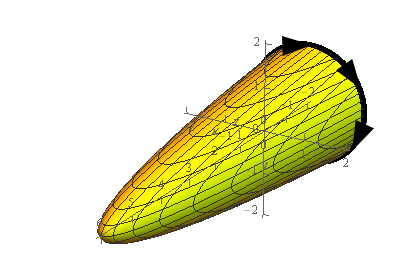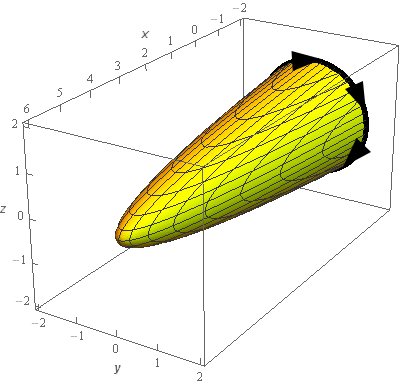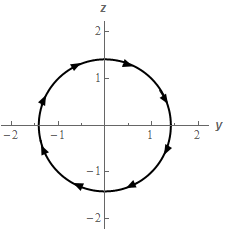Paul's Online Notes
Home / Calculus III / Surface Integrals / Stokes' Theorem
Show Mobile Notice Show All Notes Hide All Notes
Mobile Notice
You appear to be on a device with a "narrow" screen width (i.e. you are probably on a mobile phone). Due to the nature of the mathematics on this site it is best views in landscape mode. If your device is not in landscape mode many of the equations will run off the side of your device (should be able to scroll to see them) and some of the menu items will be cut off due to the narrow screen width.

### Section 17.5 : Stokes' Theorem

2. Use Stokes’ Theorem to evaluate $$\displaystyle \iint\limits_{S}{{{\mathop{\rm curl}\nolimits} \vec F\centerdot d\vec S}}$$ where $$\vec F = \left( {{z^2} - 1} \right)\,\vec i + \left( {z + x{y^3}} \right)\,\vec j + 6\,\vec k$$ and $$S$$ is the portion of $$x = 6 - 4{y^2} - 4{z^2}$$ in front of $$x = - 2$$ with orientation in the negative $$x$$-axis direction.

Show All Steps Hide All Steps

Start Solution

Let’s start off with a quick sketch of the surface we are working with in this problem.We included a sketch with traditional axes and a sketch with a set of “box” axes to help visualize the surface.

Because the orientation of the surface is towards the negative $$x$$-axis all the normal vectors will be pointing into the region enclosed by the surface. So, if we walk along the edge of the surface, i.e. the curve $$C$$, in the direction indicated with our head pointed into the region enclosed by the surface (i.e. in the same direction as the normal vectors) then our left hand will be over the region. Therefore, the direction indicated in the sketch is the positive orientation of $$C$$.

If you have trouble visualizing the direction of the curve simply get a cup or bowl and put it on its side with a piece of paper behind it. Sketch a set of axes on the piece of paper that will represent the plane the cup/bowl is sitting in front of to really help with the visualization. Then cut out a little stick figure and put a face on the “front” side of it and color the left hand a bright color so you can quickly see it. Now, on the edge of the cup/bowl/whatever you place the stick figure with its head pointing in the direction of the normal vectors (into the paraboloid/cup/bowl in our case) with its left hand over the surface. The direction that the “face” on the stick figure is facing is the direction you’d need to walk along the surface to get the positive orientation for $$C$$.

Show Step 2

We are going to use Stokes’ Theorem in the following direction.

$\iint\limits_{S}{{{\mathop{\rm curl}\nolimits} \vec F\centerdot d\vec S}} = \int\limits_{C}{{\vec F\centerdot d\vec r}}$

We’ve been given the vector field in the problem statement so we don’t need to worry about that. We will need to deal with $$C$$.

In this case $$C$$ is the curve we get by setting the two equations in the problem statement equal. Doing this gives,

$- 2 = 6 - 4{y^2} - 4{z^2}\hspace{0.25in} \to \hspace{0.25in}4{y^2} + 4{z^2} = 8\hspace{0.25in} \Rightarrow \hspace{0.25in}{y^2} + {z^2} = 2$

We will see following sketch of $$C$$ if we are in front of the paraboloid and look directly along the $$x$$-axis.One possible parameterization of $$C$$ is given by,

$\vec r\left( t \right) = \left\langle { - 2,\sqrt 2 \sin t,\sqrt 2 \cos t} \right\rangle \hspace{0.25in}\hspace{0.25in}0 \le t \le 2\pi$

The $$x$$ component of the parameterization is –2 because $$C$$ lies at $$x = - 2$$.

Show Step 3

Since we know we’ll need to eventually do the line integral we know we’ll need the following dot product.

\begin{align*}\vec F\left( {\vec r\left( t \right)} \right)\centerdot \vec r'\left( t \right) & = \left\langle {2{{\cos }^2}t - 1,\sqrt 2 \cos t - 4\sqrt 2 {{\sin }^3}t,6} \right\rangle \centerdot \left\langle {0,\sqrt 2 \cos t, - \sqrt 2 \sin t} \right\rangle \\ & = \sqrt 2 \cos t\left( {\sqrt 2 \cos t - 4\sqrt 2 {{\sin }^3}t} \right) - 6\sqrt 2 \sin t\\ & = 2{\cos ^2}t - 8\cos t{\sin ^3}t - 6\sqrt 2 \sin t\\ & = \left( {1 + \cos \left( {2t} \right)} \right) - 8\cos t{\sin ^3}t - 6\sqrt 2 \sin t\end{align*}

Don’t forget to plug the parameterization of $$C$$ into the vector field!

We also did a little simplification on the first term with an eye towards the integration.

Show Step 4

Okay, let’s go ahead and evaluate the integral using Stokes’ Theorem.

\begin{align*}\iint\limits_{S}{{{\mathop{\rm curl}\nolimits} \vec F\centerdot d\vec S}} & = \int\limits_{C}{{\vec F\centerdot d\vec r}}\\ & = \int_{0}^{{2\pi }}{{\left( {1 + \cos \left( {2t} \right)} \right) - 8\cos t{{\sin }^3}t - 6\sqrt 2 \sin t\,dt}}\\ & = \left. {\left( {t + \frac{1}{2}\sin \left( {2t} \right) - 2{{\sin }^4}t + 6\sqrt 2 \cos t\,dt} \right)} \right|_0^{2\pi } = \require{bbox} \bbox[2pt,border:1px solid black]{{2\pi }}\end{align*}